# Karat

The karat is a unit of purity that is somewhat similar to fineness for gold alloys. According to Scientific American, the karat is 1/24 part pure gold by weight.

Weight is really just a force, a specific force comprised of the acceleration due to gravity multiplied by the mass of an object. If we simply cancel the acceleration due to gravity to eliminate weight, we are left with mass. Thus, karat can be used to describe how much pure gold mass there is in an object.

When using the karat to establish value, there is little regard for what the rest of the object contains. And so, karat is strictly the mass of pure gold in an object and what twenty-four part fraction it has in relation to the rest of the object.

Since karat is a ratio of masses based upon twenty-four equal-mass parts, we have the gold portion over the total portions, multiplied by twenty-four as the number of parts out of twenty-four parts. For example, if we had one portion gold and two other portions copper, we’d have one divided by “one plus two”, making the fraction one third.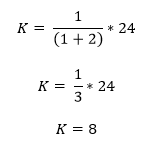One third multiplied by twenty-four is eight. Therefore, in this example, eight parts in twenty-four are pure gold by mass. So, this would be an 8 karat gold item. The karat number 8 only really becomes useful as a decimal when it used in conjunction with the 1/24th part: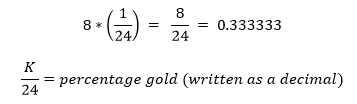For 8 karat value, we don’t factor in what the other sixteen parts of the object are worth. We strictly value this object on its gold content. However, in this case and in most cases, the particle’s total volume would be greater than if the particle were pure gold. Since we have an alloy, we need to factor in the density of copper or silver in order to properly determine how much total volume there should be for this particle specifically.

Likewise, if there was no copper or silver in the particle at all, we’d have one portion gold divided by “zero portions alloyed material plus one portion gold”, making the fraction one over one, or simply just one.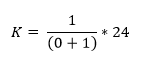One multiplied by twenty-four is twenty-four.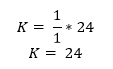And so, twenty-four parts in twenty-four are pure gold, therein 24 karat gold (24/24 which is 1 as a decimal).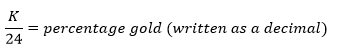This sort of particle would have the highest density and the lowest volume, since it is pure gold (100%). Occasionally, in the western United States, purity hovers near 19 karat (80%) for native gold. Here is an example of documented gold fineness for a given area report, and map.